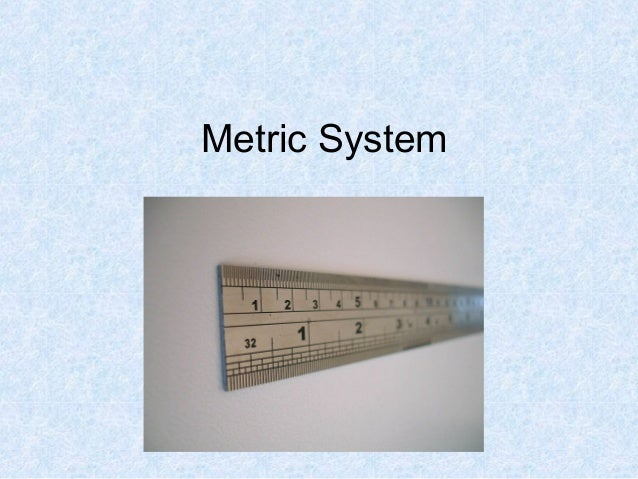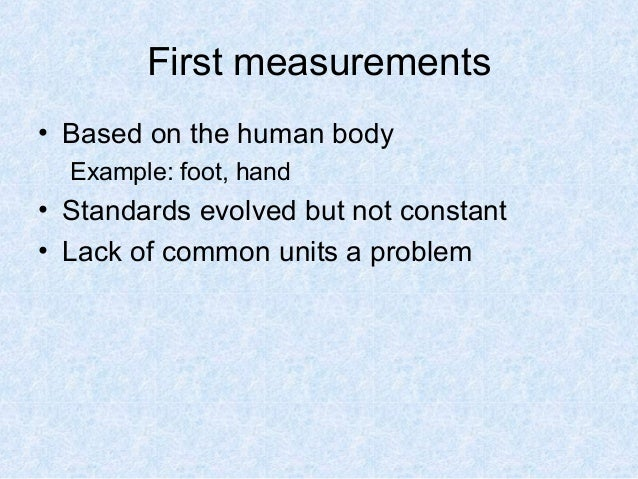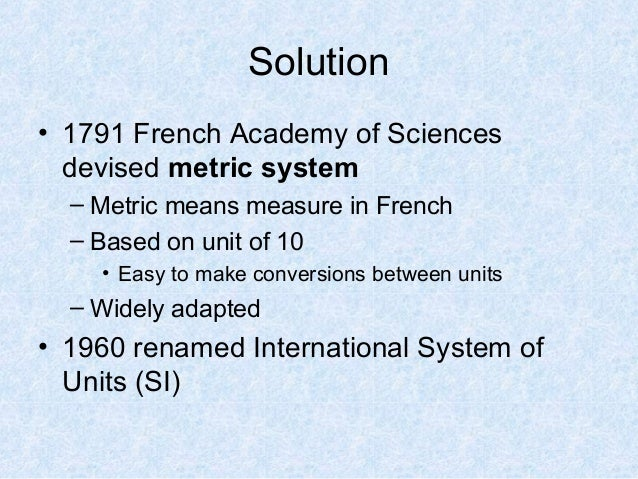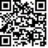Successfully reported this slideshow.Upcoming SlideShare
×

ofUpcoming SlideShare
Food choice tz
Next

2 Likes

Share

# Metric System

Biology I Lab instructions: Metric System

See all

See all

### Metric System

1. 1. Metric System
2. 2. First measurements • Based on the human body Example: foot, hand • Standards evolved but not constant • Lack of common units a problem
3. 3. Solution • 1791 French Academy of Sciences devised metric system – Metric means measure in French – Based on unit of 10 • Easy to make conversions between units – Widely adapted • 1960 renamed International System of Units (SI)
4. 4. Metric Units Unit Metric measure Abbreviation Length Meter m Volume Liter L Mass Gram g Temperature Celcius C
5. 5. Metric prefixes we’ll use Prefix Symbol Multiplier Notation nano n 0.000000001 10-9 micro µ 0.000001 10-6 milli m 0.001 10-3 centi c 0.01 10-2 Base unit g, m, L 1 100 kilo k 1000 103
6. 6. Metric System • An easy way to move within the metric system is by moving the decimal point one place for each “step” desired Example: change meters to centimeters 1 meter = 10 decimeters = 100 centimeters or 1.00 meter = 10.0 decimeters = 100. centimeters kilo hecto deca meter liter gram deci centi milli
7. 7. Metric System • If you move to the left in the diagram, move the decimal to the left • If you move to the right in the diagram, move the decimal to the right kilo hecto deca meter liter gram deci centi milli
8. 8. Metric conversion examples 15.2 millimeters = _________________ meters 13 centigrams= __________________ micrograms 9.74 liters = __________________ milliliters 8051 nanometers = ______________ micrometers
9. 9. Scientific Notation • You can work backwards from scientific notation – The number in the superscript position of the 10 tells you how many places to move the decimal and in what direction 1 x 103 = 1000 4.7 x 105 = 470000 3.2 x 10-3 = 0.0032
10. 10. Length: Meter (cm, mm) • Based on the physical measure on the earth – Length from the North Pole to the Equator divided into 10 million parts • Measure with a ruler or tape measurer
11. 11. Mass/Weight: Grams (mg) • The quantity of matter in a material • Gram is typical unit used in the laboratory • Measure with scale or balance
12. 12. Volume: Liter (ml) • Space occupied by a unit – 3 dimensional • Units are cubed • ml=cm3 • Typically used to measure liquids • Meniscus: curvature of water
13. 13. Micropipette • Tool used to pipette small volumes of liquids • Measure in microliters (10-6 ) • Will use this tool again later in the semester
14. 14. Temperature: Celsius (C°) • Measure of concentration of heat • The average amount of motion per molecule 0°C=freezing point of H2O 100°C=boiling point of H2O To convert: F to C: C = ( F - 32) / 1.8 C to F: F = C × 1.8 + 32
•#### AmberMarquez2

Dec. 3, 2021
•#### MarkAngelPermejo

Sep. 27, 2019

Total views

32,124

On Slideshare

0

From embeds

0

Number of embeds

27,764

75

Shares

0